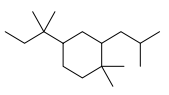# Problem: Please answer the following questions for the compound given below. a) Provide a name b) How many methine groups are there? c) Give the molecular formula for this compound d) For the number of Cs that you gave in the moleular formula for this problem, what is the maximum possible number of Hs? e) How many secondary Hydrogens are there? f) How many quaternary Cs are there?

🤓 Based on our data, we think this question is relevant for Professor Minto's class at IUPUI.

###### Problem Details

a) Provide a name

b) How many methine groups are there?

c) Give the molecular formula for this compound

d) For the number of Cs that you gave in the moleular formula for this problem, what is the maximum possible number of Hs?

e) How many secondary Hydrogens are there?

f) How many quaternary Cs are there?What scientific concept do you need to know in order to solve this problem?

Our tutors have indicated that to solve this problem you will need to apply the Naming Cycloalkanes concept. You can view video lessons to learn Naming Cycloalkanes. Or if you need more Naming Cycloalkanes practice, you can also practice Naming Cycloalkanes practice problems.

What is the difficulty of this problem?

Our tutors rated the difficulty ofPlease answer the following questions for the compound given...as medium difficulty.

How long does this problem take to solve?

Our expert Organic tutor, Chris took 12 minutes and 51 seconds to solve this problem. You can follow their steps in the video explanation above.

What professor is this problem relevant for?

Based on our data, we think this problem is relevant for Professor Minto's class at IUPUI.